# 9Time Series

Almost all the data we use here is associated with a particular point in time, like a price of a stock on a given day. That is called a time series. However, it is difficult to work with time series as one has to keep track of the day month and year, know about leap years, time zones, holidays and other market closures. Consequently, it is usually best to work with data as if it were not a time series, and only turning it into a time series when needed, typically for plotting, reporting and aggregation.

Most of financial applications involve working with dates. It can be monthly, weekly, daily, or even intraday data. Storing data as text is not helpful since we cannot order or subset it easily.

R has a specific data type called `Date`. In this section we will explore some packages that will help us to work with `Date` objects.

## 9.1 Libraries

``````suppressPackageStartupMessages(library(lubridate))
suppressPackageStartupMessages(library(zoo))``````

``````load('data/data.RData')
sp500=data\$sp500
sp500tr=data\$sp500tr
Price=data\$Price
Return=data\$Return
Ticker=data\$Ticker
names(data)``````
`````` "Return"          "Price"           "UnAdjustedPrice" "sp500"
 "sp500tr"         "Ticker"         ``````

## 9.3 Plotting time series

Start by plotting the SP-500 with the default plot:

``````par(mar=c(3,3,0,0))
plot(sp500\$price)``````It is quite ugly, and we can easily improve it a bit:

``````par(mar=c(2,4.2,1,0))
plot(sp500\$price,
type='l',
lwd=2,
col='blue',
las=1,
bty='l',
xlab="day",
ylab='price',
main="The SP-500 index"
)``````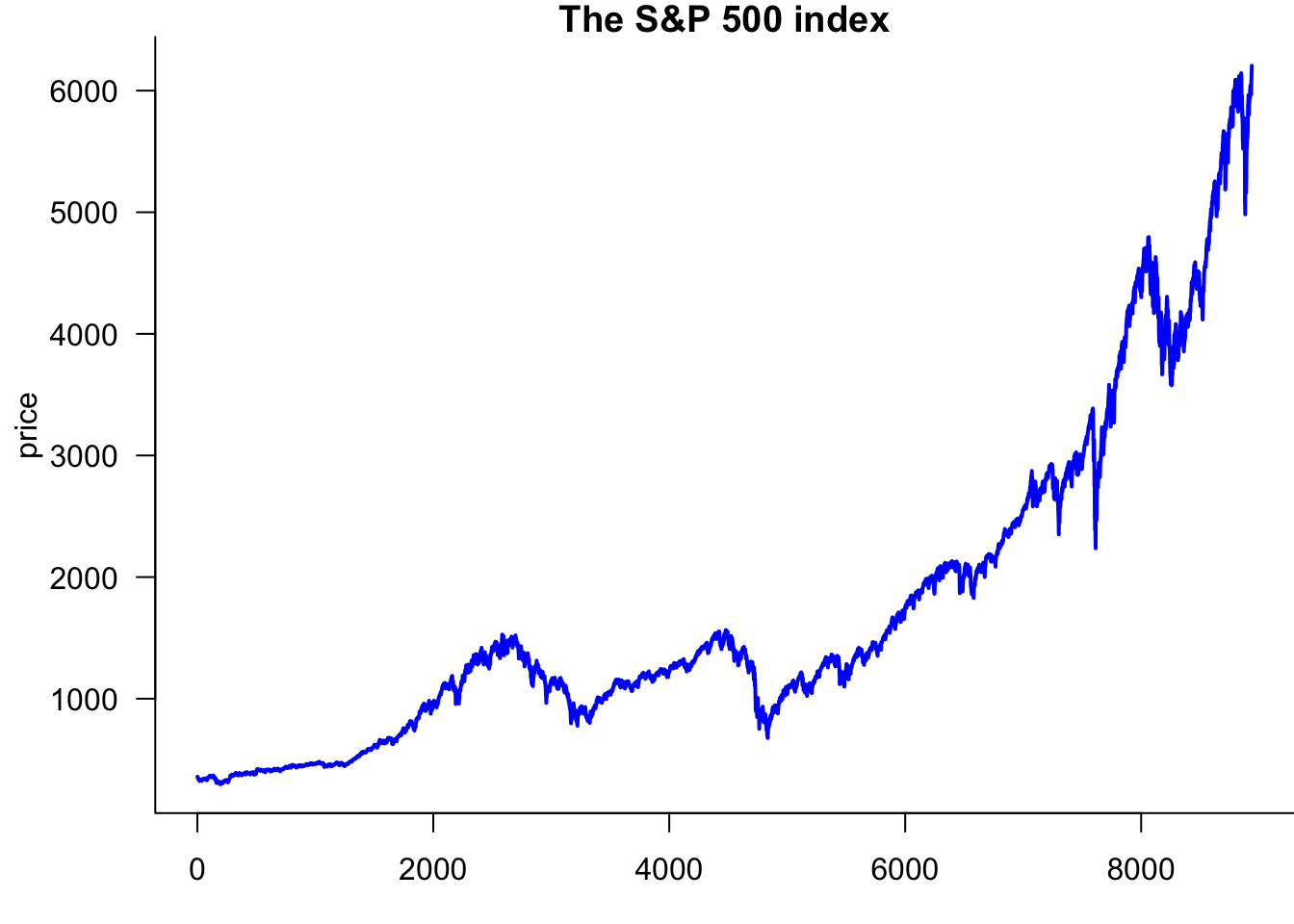## 9.4`lubridate`

We use the `lubridate` package to convert numbers and strings into dates. `ymd()`stands for year-month-day. If we have data with the American date convention, we can use `mdy()`, and in some cases we have `ymd()` formatted dates.

`lubridate` handles both string labels, JAN and integer `01`.

``ymd("20200110")``
`` "2020-01-10"``
``class(ymd("20200110"))``
`` "Date"``
``ymd("2015JAN11")``
`` "2015-01-11"``
``class(ymd("20200110"))``
`` "Date"``
``ymd("04-MAR-5")``
`` "2004-03-05"``
``class(ymd("04MAR5"))``
`` "Date"``
``dmy("1/june/2019")``
`` "2019-06-01"``
``class(dmy("1/june/2019"))``
`` "Date"``
``dmy("28-december-14")``
`` "2014-12-28"``
``class(dmy("28-december-14"))``
`` "Date"``

## 9.5 Plotting with dates

We can make a proper date column for the SP-500

``````sp500\$date.ts=ymd(sp500\$date)
tail(sp500,2)``````
``````         date   price            y    date.ts
5034 20221229 3849.28  0.017310619 2022-12-29
5035 20221230 3839.50 -0.002543968 2022-12-30``````

That allows us to make a time series plot.

``````par(mar=c(2,4,1,0))
plot(sp500\$date.ts,sp500\$price,
type='l',
lwd=2,
col='blue',
las=1,
bty='l',
xlab="Day",
ylab='Price',
main="The SP-500 index"
)``````You can make it a log plot

``````par(mar=c(2,4,1,0))
plot(sp500\$date.ts,sp500\$price,
type='l',
lwd=2,
col='blue',
las=1,
bty='l',
xlab="day",
ylab='price',
main="The SP-500 index",
log='y'
)``````## 9.6 The `zoo` package

This package functions allows us to work with ordered date indexed observations.

``````sp500\$y.ts = zoo(sp500\$y, order.by = sp500\$date.ts)
sp500\$price.ts = zoo(sp500\$price, order.by = sp500\$date.ts)
class(sp500\$y.ts)``````
`` "zoo"``
``head(sp500\$y.ts)``
``````   2003-01-03    2003-01-06    2003-01-07    2003-01-08    2003-01-09
-0.0004841496  0.0222255557 -0.0065661110 -0.0141857185  0.0192005899
2003-01-10
0.0000000000 ``````

And then we can plot it directly as time series.

``````par(mar=c(2,4,2,0))
plot(sp500\$y.ts, main="S&P500 Daily Return", ylab="Return")``````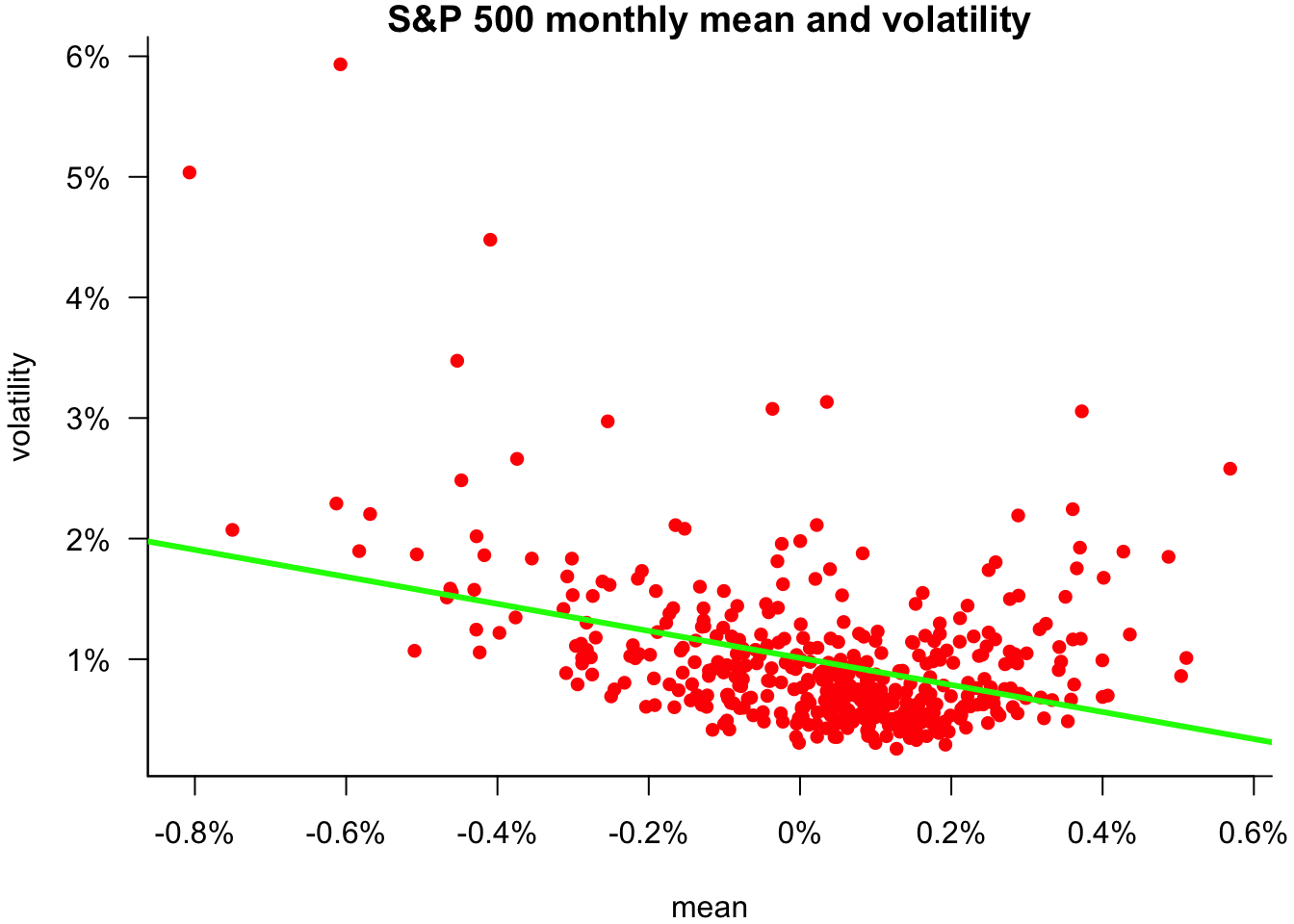We can do useful things with zoo data.

### 9.6.1`lag` function

This function allows us to take the lag or leads of a time series object. The syntax is: `lag(x, k, na.pad = F)`, where:

• x, a time series object to lag
• k, number of lags (in units of observations); could be positive or negative (if negative, k is number of forward lags)
• `na.pad`, adds `NAs` for missing observations if `TRUE`
``head(sp500\$y.ts)``
``````   2003-01-03    2003-01-06    2003-01-07    2003-01-08    2003-01-09
-0.0004841496  0.0222255557 -0.0065661110 -0.0141857185  0.0192005899
2003-01-10
0.0000000000 ``````
``head(lag(sp500\$y.ts, k = 2))``
``````  2003-01-03   2003-01-06   2003-01-07   2003-01-08   2003-01-09   2003-01-10
-0.006566111 -0.014185718  0.019200590  0.000000000 -0.001413291  0.005812968 ``````

### 9.6.2`diff` function

Takes the lagged difference of a time series. Syntax: `diff(x, lag, differences, na.pad = F)`, where:

• x = a time series object
• lag = number of lags(in unit of observations)
• differences = the order of the difference
``head(diff(sp500\$y.ts, lag = 1, na.pad = TRUE))``
``````  2003-01-03   2003-01-06   2003-01-07   2003-01-08   2003-01-09   2003-01-10
NA  0.022709705 -0.028791667 -0.007619607  0.033386308 -0.019200590 ``````

### 9.6.3 The `window` function

We can use the `window()` function to subset a zoo object to a given time period. For example, let’s say we are interested in the returns during the Covid-19 crisis:

``````par(mar=c(2,4,1,0))
sub_y.ts = window(sp500\$y.ts, start = ymd("20200201"), end = ymd("20200401"))
plot(sub_y.ts,
main = "Returns in Covid",
xlab = "Date",
ylab = "Returns",
col = "mediumblue",
lwd=2
)``````### 9.6.4 Aggregate

We quite often need to aggregate time series data. Perhaps we want to calculate end of month prices or realised monthly variance. That is easily done with the `aggregate` function

``````p.monthly=aggregate(sp500\$price.ts,as.yearmon,tail,1)
``````Jan 2003 Feb 2003 Mar 2003 Apr 2003 May 2003
855.70   841.15   848.18   916.92   963.59 ``````
``````realized.variance=aggregate(sp500\$y.ts,as.yearmon,sd)
``````  Jan 2003   Feb 2003   Mar 2003   Apr 2003   May 2003
0.01435287 0.01188719 0.01747653 0.01169788 0.01026536 ``````
``p.monthly.mean=aggregate(sp500\$y.ts,as.yearmon,mean)``
``````par(mar=c(4,4,1,0.6))
plot(p.monthly.mean,realized.variance,
bty='l',
main="SP-500 monthly mean and volatility",
col='red',
pch=16,
xlab="mean",
ylab="volatility",
xaxt='n',
yaxt='n'
)
w=pretty(p.monthly.mean)
axis(1,w,label=paste0(100*w,"%"))
w=pretty(realized.variance)
axis(2,w,label=paste0(100*w,"%"),las=1)
regression_line=lm(realized.variance ~ p.monthly.mean)
abline(regression_line,col='green',lwd=3)``````## 9.7 Multivariate plots

We use the `matplot` command for many assets. Call the list of assets `Assets`:

``````par(mar=c(2,4,0,0))
matplot(Price[,Ticker])``````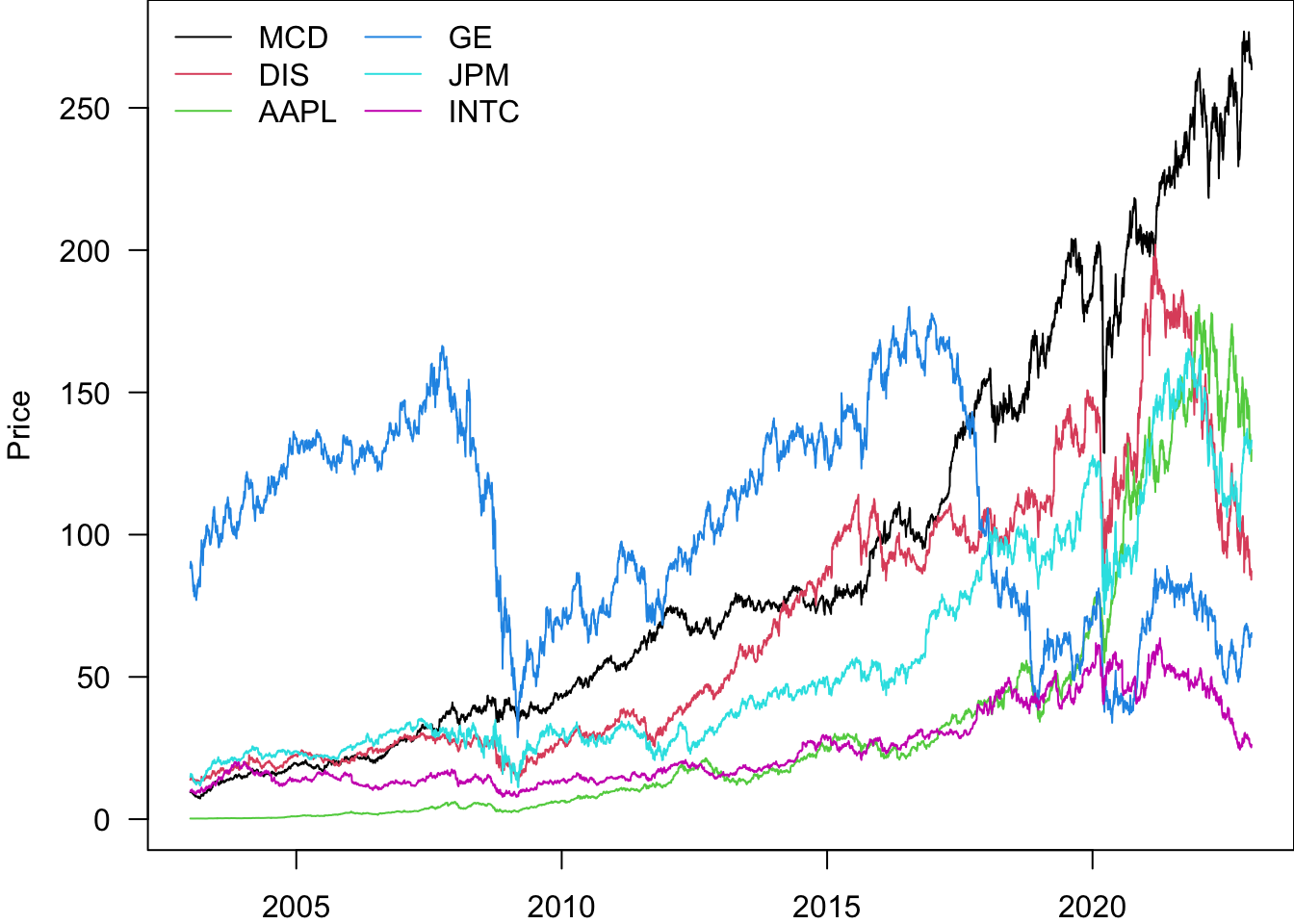This is quite ugly, and can be made to look better

``````par(mar=c(2,4,0,0))
matplot(
Price[,Ticker],
type='l',
lty=1,
ylab='Price'
)``````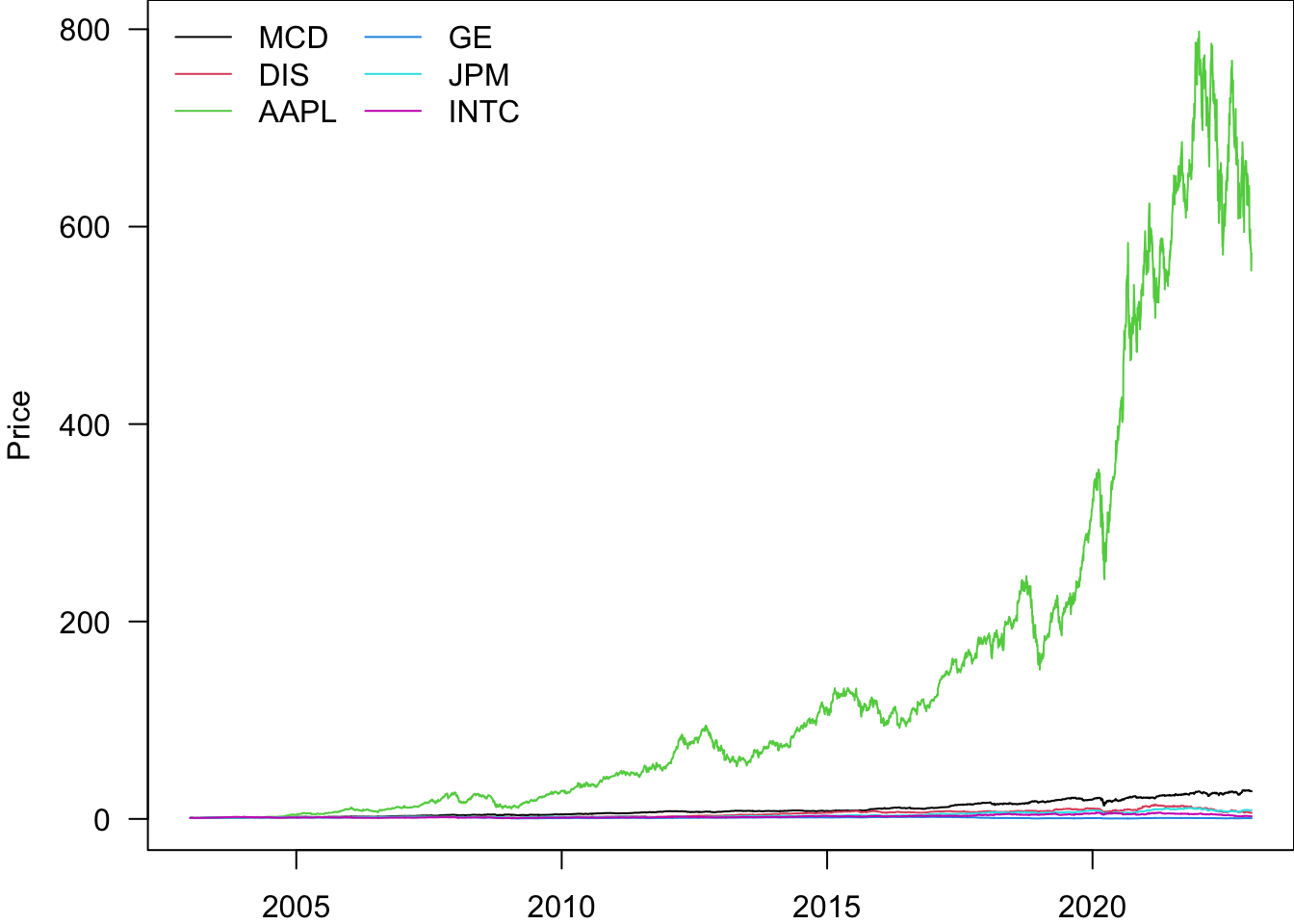We can add a date to it in the same way as before.

``````Price\$date.ts=ymd(Price\$date)
Return\$date.ts=ymd(Return\$date)
``````par(mar=c(2,4,0,0))
matplot(
Price\$date.ts,
Price[,Ticker],
type='l',
lty=1,
ylab='Price'
)``````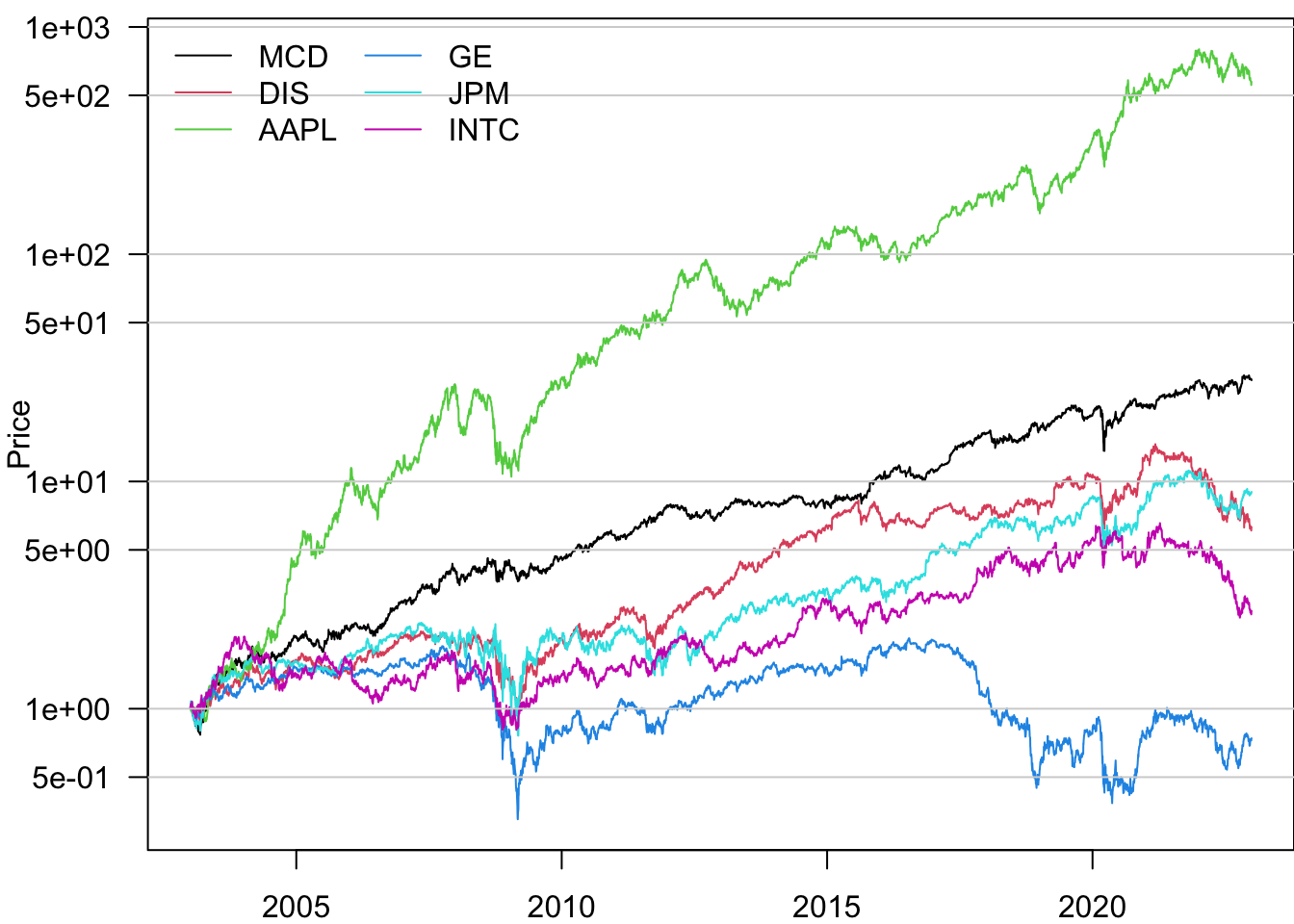We can put a legend on the plot

``````par(mar=c(2,4,0,0))
matplot(
Price\$date.ts,
Price[,Ticker],
type='l',
lty=1,
ylab='Price',
col=1:6,
las=1
)
legend("topleft",legend=Ticker,lty=1,col=1:6,bty='n',ncol=2)``````In order to compare the performance of the stocks, we can re-normalise them to start at 1

``````par(mar=c(2,4,0,0))
pn=Price
for(i in Ticker){
pn[[i]]=pn[[i]]/pn[[i]]
}
matplot(
pn\$date.ts,
pn[,Ticker],
type='l',
lty=1,
ylab='Price',
col=1:6,
las=1
)
legend("topleft",legend=Ticker,lty=1,col=1:6,bty='n',ncol=2)````````rbind(head(pn,2),tail(pn,1))``
``````         date     AAPL      DIS        GE     INTC      JPM       MCD
2    20030103   1.0000 1.000000 1.0000000 1.000000 1.000000  1.000000
3    20030106   1.0000 1.051264 1.0255903 1.038697 1.078639  1.032873
5035 20221230 572.7678 6.279998 0.7411147 2.692354 8.998689 27.909514
date.ts
2    2003-01-03
3    2003-01-06
5035 2022-12-30``````

``````par(mar=c(2,4,0,0))
pn=Price
for(i in Ticker){
pn[[i]]=pn[[i]]/pn[[i]]
}
matplot(
pn\$date.ts,
pn[,Ticker],
type='l',
lty=1,
ylab='Price',
col=1:6,
las=1,
log='y'
)
legend("topleft",legend=Ticker,lty=1,col=1:6,bty='n',ncol=2)``````And put gridlines on it.

``````par(mar=c(2,4,0,0))
pn=Price
for(i in Ticker){
pn[[i]]=pn[[i]]/pn[[i]]
}
matplot(
pn\$date.ts,
pn[,Ticker],
type='l',
lty=1,
ylab='Price',
col=1:6,
las=1,
log='y'
)
for(i in c(0.5,1,5,10,50,100,500,1000))
segments(pn\$date.ts-days(500),i,tail(pn\$date.ts,1)+days(500),i,col="lightgray")

legend("topleft",legend=Ticker,lty=1,col=1:6,bty='n',ncol=2)``````So based on this, it appears the best performing stock is AAPL.

## 9.8 Saving time series information

We can now save the data with the time series embedded. For clarity, we repeat the time series calculations here, so we have a summary of them. The output file is called `data.ts.Rdata`.

``````load('data/data.RData')
data.ts=data

data.ts\$sp500\$date.ts=ymd(sp500\$date)
data.ts\$sp500\$y.ts = zoo(sp500\$y, order.by = data.ts\$sp500\$date.ts)
data.ts\$sp500\$price.ts = zoo(sp500\$price, order.by = data.ts\$sp500\$date.ts)

data.ts\$sp500tr\$date.ts=ymd(sp500tr\$date)
data.ts\$sp500tr\$price.ts = zoo(sp500tr\$price, order.by = data.ts\$sp500tr\$date.ts)
data.ts\$sp500tr\$y.ts = zoo(sp500tr\$y, order.by = data.ts\$sp500tr\$date.ts)

data.ts\$Price\$date.ts=ymd(Price\$date)
data.ts\$Return\$date.ts=ymd(Return\$date)